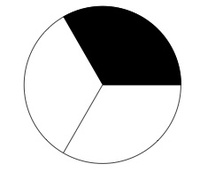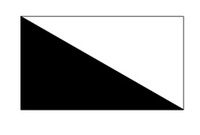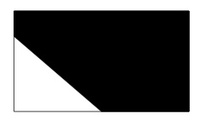# Which Pictures Represent One Half?

Alignments to Content Standards: 2.G.A.3

1. Which pictures show one half of the shape shaded? Explain.

1.2.3.4.2. Is more or less than one half of the shape shaded in (ii)? Explain.
3. Is more or less than one half of the shape shaded in (iv)? Explain.

## IM Commentary

The purpose of this task is for students to see different ways of partitioning a figure into two or more equal shares, by which we mean decomposing the figure into "pieces" with equal area. In third grade, students should be able to reason in general about the relative size of unit fractions based on the idea that they are created by partitioning a whole into a certain number of equal shares; in second grade they begin to build this understanding by working with geometric figures that represent the unit fractions $\frac12, \frac13,$ and $\frac14$.

For picture (i), students will intuitively see that the circle is composed of two pieces with equal area and that one half of the circle is shaded. From here, it is not a very big stretch for students to see that the circle in (ii) is composed of three pieces with equal area and that one of these is shaded and that this is less than one half.

It is less intuitive for students to think of the shaded region in picture (iii) as representing one half. This is in part due to the fact that the pieces are less symmetrical, and the transformation needed to show they are the same size and same shape is harder to visualize for some students. Students struggling with this would benefit from having some tracing paper that would allow them to physically demonstrate that one can be rotated to exactly match up with the other.

For picture (iv), students need to compare areas of different shapes and decide whether the shaded area is more or less than one half. If some students suggest that one half of this picture is shaded, the teacher may need to remind student that the shaded and unshaded regions must have equal area.

The Standards for Mathematical Practice focus on the nature of the learning experiences by attending to the thinking processes and habits of mind that students need to develop in order to attain a deep and flexible understanding of mathematics. Certain tasks lend themselves to the demonstration of specific practices by students. The practices that are observable during exploration of a task depend on how instruction unfolds in the classroom. While it is possible that tasks may be connected to several practices, only one practice connection will be discussed in depth. Possible secondary practice connections may be discussed but not in the same degree of detail.

This particular task helps illustrate Mathematical Practice Standard 3, Construct viable arguments and critique the reasoning of others. This type of exercise lays the foundation for the art of explanation leading to “critiquing the reasoning of others.” Before students can critique the reasoning of others, they must feel comfortable in supporting their own thinking with evidence. For example, a second grader might offer the explanation, “I know that the first circle is divided into two equal parts so the shaded part is one half of the circle.” Pictures iii and iv may be more challenging for students to explain because the pieces in each shape are either less symmetrical or are completely different.  So the teacher might ask the class, “How might you show if the two pieces are equal?” Also, the teacher can easily extend the classroom discussion regarding these pictures to the next level by asking, “Do you agree with Student A’s explanation and why?” This type of math talk in the classroom is built through collaborative problem solving and dialogue.

## Solution

1. One half of the circle is shaded because the shaded region and the unshaded region have the same shape and size, making each one half of the full circle. This is perhaps the picture most frequently associated with the words "half of a circle" or "semi-circle."
2. Less than one half of this circle is shaded. Visually, the shaded area is far less then the unshaded area while the two would be equal if the shaded area represented one half of the circle. Mathematically, the shaded area represents one third of the circle since one of three equal pieces is shaded. One way to see this is to note that if a cookie is divided into 3 equal shares, each share is less than if the same cookie is divided into two equal shares.
3. One half of this rectangle is shaded. This can be seen geometrically as the shape and size of the black triangle is identical to the shape and size of the white triangle, making each one half of the whole rectangle. Concretely, if the rectangle is cut in half and then the white triangle is rotated by $180$ degrees about the center of the rectangle, then it will lie precisely on top of the black triangle.
4. More than one half of this rectangle is shaded. Visually the black shaded piece is far larger than the white unshaded piece and so this picture does not show one half.

1. In picture (ii), the shaded area is equal to each of the two unshaded pieces and so the unshaded area is larger than the shaded area (twice as large). So the shaded area represents less than one half.

2. In picture (iv), the shaded part of the rectangle is larger than the unshaded part and so the shaded area is more than one half of the area of the rectangle. Unlike picture (ii) which represents one third, however, the lower picture does not provide enough information to conclude precisely what fraction of the rectangle is shaded.